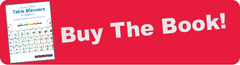# 1 - The Ross Periodic Table

## This periodic table is designed for work!

### 1.   Extremely Easy to Learn

Start with a blank periodic table, with the same number of rows and columns as above. These are the main block elements. Your students can reproduce the rest of the table in five simple steps.

1. On Row 2, make a horizontal series of eight small circles to represent the atomic cores
2. Number the circles consecutively from 1+ to 8+ to represent the core charge
3. Draw a large circle around the first core to represent the valence orbit
4. Draw consecutively smaller valence circles from 1+ to 8+ atomic cores
5. Draw the correct number of valence electrons around the valence orbit. (The number of valence  electrons equals the core charge.)

That's it! Rows three and four are exactly the same, except that each valence shell is slightly larger in the lower rows.

### 2.   Only Three Salient FeaturesThe core charge, the radius, and the valence occupation are the three features that students use to make judgements about the chemical behavior of the elements.

They are also the three most visible features of each diagram.

Students don't have to wade through the fifty or so details of a Bohr-Rutherford atom.

### 3.   Students Intuitively Understand It.

To understand its chemistry, a student needs to consider only three facts about each atom.

1. The stronger the core charge, the more the valence electrons are attracted.
2. The smaller the radius, the stronger the attractive force.
3. An atom with a full valence shell cannot attract and hold any more electrons.
It's easy to see that an atom with a large core charge, a small radius, and one or more vacancies can attract and hold electrons from other atoms.

It really is that easy. These three facts are based upon students' intuitive schematic reasoning patterns. Intuitive schemata are very deeply part of us. Even cats, dogs and birds follow similar patterns!

### 4.   Now Use the Table!1. Walk your students through the table, pointing out core charge.
2. Remove the table from view.
3. Ask them to make their own copy of the table on a piece of paper.

Most students will be able to draw the atomic cores with their positive charges, and the relative radius of each atom. Those few students who don't get it the first time, will probably be successful on the second try.

Lesson 1 - Learn the three salient features of each atom, and follow how they change across the periodic table.

Lesson 2 - Learn how students can make accurate predictions, with nearly no instruction!

Lesson 3 - Take a closer look at the elements of the second row.

Lesson 4 - Origins of the Ross model of the atom

Lesson 5 - Covalent bonding

Lesson 6 - Ionic Bonding

Lesson 7 - A post-modern model of science.

Lesson 8 - Learning with IntuitivScience.